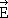# How to find angle Electric field makes to x-axis?

• Dana Fishel
This way, you won't have to rely on your calculator's arctan function to give you the correct answer.

## Homework Statement

Find the angle thatmakes with the positive x axis:
(Consider vectors above the x-axis to make positive angles and vectors below the x-axis to make negative angles.)

## Homework Equations

pheta = arctan (Ex/Ey)
tan(pheta) = Ex/Ey

## The Attempt at a Solution

I already found that Ex= -2 N/C and Ey= -2 and IEI = 2.83 N/C
So I tried pheta = arctan (-2/-2) = 45 degrees but it's still marked incorrect.

Hello and welcome to PF!

Try to decide which quadrant of the x-y plane the E vector points in. Note the signs of the x and y components.

The arctan function of many calculators will only return angles between -90 and + 90 degrees (i.e., 1st and 4th quadrants).

I wasn't sure about this so I tried +45 degrees and -45 degrees, but those are wrong. Would subtracting from 180 or 360 help?

Did you make a sketch showing the E vector in the x-y plane? Can you see from the sketch the answer for the angle? To get the answer, you shouldn't have to use your calculator.

I drew one, but can't get it to upload. So since both values are negative, it would be in quadrant 3, but I'm still confused about finding pheta.
Since Ex and Ey make a triangle with the y and x-axis and we want to use tan, is pheta 90 degrees?

Never mind. I tried doing pheta = arcos of (-2/2.83) = -135degrees (since quadrant 3)
and that's correct, but I don't really get why it works...

Is it because the angle you get is the outside angle to the triangle? (Sorry this sounds really weird without the picture.)

Thank you for your help though!

Last edited:
The arctan function cannot tell how the individual signs of any vector components are distributed between the numerator or denominator of the ratio that forms its argument. It only sees the result of any such division.

A positive valued argument may indicate a vector in the first quadrant (two positive values for the ratio) or in the third quadrant (two negatives yielding a positive valued argument). A negative argument could mean a vector in either the 2nd or 4th quadrant.

Because of this ambiguity which cannot be avoided with a single function argument, the arctan function is constrained to only return angles in the 1st and 4th quadrants. It's up to you to sort out the actual quadrant and translate the result accordingly.

Many calculators and programming languages also have the atan2() function which takes two arguments, one for the numerator and one for the denominator of the ratio. It then has access to the individual signs of the vector components and will return a result assigned to the correct quadrant.

Suppose you had a vector with x component +2 and y component +2. If you use the arctan function on your calculator to find the angle, you get ##arctan(\frac{+2}{+2}) = arctan(1)= 45^o##. This is correct for the angle as measured counterclockwise from the positive x axis.

In your case, you have a vector with x component -2 and y component -2. Your calculator gives ##arctan(\frac{-2}{-2}) = arctan(1)= 45^o##. This is not the correct angle as measured from the positive x axis. You can see why your calculator can't handle this correctly since it naturally treats ##\frac{-2}{-2}## as identical to ##\frac{+2}{+2}##. In other words, your calculator's arctan function can't tell the difference between a vector in the first quadrant and a vector in the third quadrant. Likewise, it can't distinguish between a vector in the 4th quadrant and vector in the 2nd quadrant.